# Exam-Style Questions.

## Problems adapted from questions set for previous Mathematics exams.

### 1.

GCSE Higher

The midnight train to Georgia is not the most reliable. The probability that the train will be late on any day is 0.35

(a) Complete the probability tree diagram for Monday and Tuesday.

Monday                 Tuesday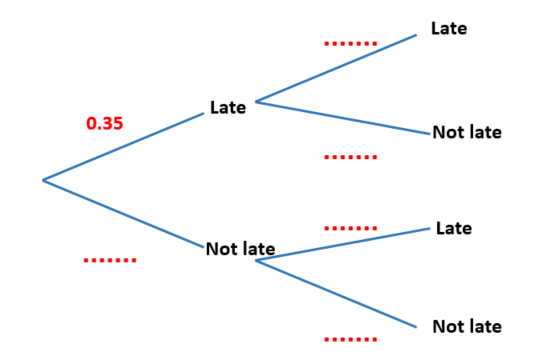(b) Work out the probability that the train will be late on at least one of these two days.

### 2.

GCSE Higher

A driving test has two sections, practical(p) and theory(t). One day everyone who took the test passed at least one section. 77% passed the practical section and 81% passed the theory section.

(a) Represent this information on a Venn diagram showing the percentage of candidates in each section of the diagram.One person is chosen at random from all the people who took the test that day. What is the probability that this person:

(b) passed the practical section, given that they passed the theory section,

(c) passed the theory section, given that they passed only one section?

### 3.

GCSE Higher

The Venn diagram represents a collection of 40 books on sale in an online store.

P represents the books available in paperback format.

D represents the books available in digital format.(a) One book from the collection is picked at random. Work out the probability that the book is available in digital format.

(b) One of the books that is available in paperback format is picked at random. Work out the probability that this book is also available in digital format.

### 4.

IB Studies

Twenty nine children went to a zoo to photograph some animals. The numbers of children who photographed Lions(L), Monkeys(M) and Zebras (Z) are given as follows:

• 6 of the children photographed all three
• 9 photographed Lions and Zebras
• 2 photographed Monkeys and Lions but not Zebras
• 3 photographed Lions only
• 4 photographed Monkeys only
• 10 photographed Zebras only

(a) Draw a Venn diagram to show this information.

All of the children photographed at least one of the three types of animal.

(b) Find the number of children that photographed Monkeys and Zebras but not Lions.

(c) Calculate the probability that a child chosen at random from the group photographed a Lion.

(d) Calculate the probability that a child chosen at random from the group photographed only one of the three types of animal.

(e) Calculate the probability that a child chosen at random from the group photographed only Lions, given that they saw only one of the three types of animal.

(f) If a child chosen at random from the group photographed the Lions, find the probability that they also photographed the Monkeys.

### 5.

IB Studies

Neal is attending a Scout jamboree in Japan. He has both boots and trainers to wear. He also has the choice of wearing a cap or not.

The probability Neal wears boots is 0.4. If he wears boots, the probability that he wears a cap is 0.7.

If Neal wears trainers, the probability that he wears a cap is 0.25.

The following tree diagram shows the probabilities for Neal's clothing options at the jamboree.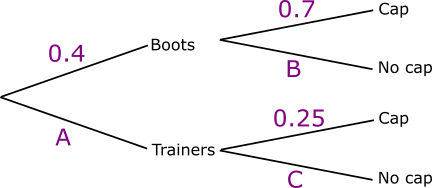(a) Find the value of A.

(b) Find the value of B.

(c) Find the value of C.

(d) Calculate the probability that Neal wears trainers and no cap.

(e) Calculate the probability that Neal wears no cap.

(f) Calculate the probability that Neal wears trainers given that he is not wearing a cap.

(g) Calculate the probability that Neal wears boots on the first two days of the jamboree.

(h) Calculate the probability that Neal wears boots on one of the first two days, and trainers on the other.

### 6.

IB Analysis and Approaches

Let A and B be events such that $$P (A) = 0.45$$ , $$P (B) = 0.35$$ and $$P (A \cup B) = 0.5$$.

Find $$P (A \mid B)$$.

### 7.

IB Analysis and Approaches

Bag A contains 6 green tickets and 5 blue tickets.

Bag B contains 4 green tickets and 1 blue tickets.

(a) A bag is chosen at random and a ticket is drawn. Find the probability that the ticket is green.

Let J be the event that "bag A is chosen" and let K be the event that "a green ticket is drawn".

(b) Determine whether events J and K are independent.

### 8.

IB Standard

The Venn diagram below shows the events $$A$$ and $$B$$, and the probabilities p, q and r.

It is known that $${\rm{P}}(A) = 0.43$$ , $${\rm{P}}(B) = 0.62$$ and $${\rm{P}}(A \cap B) = 0.27$$ .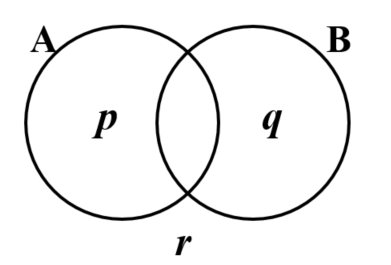(a) Calculate the value of p.

(b) Calculate the value of q.

(c) Calculate the value of r.

(d) Find the value of $${\rm{P}}(A|B')$$

(e) Hence, or otherwise, show that the events $$A$$ and $$B$$ are not independent.

### 9.

IB Studies

Julie chooses a cake from a yellow box on a shelf. The box contains two chocolate cakes and three plain cakes. She eats the cake and chooses another one from the box. The tree diagram below represents the situation with the four possible outcomes where C stands for chocolate cake and P for plain cake.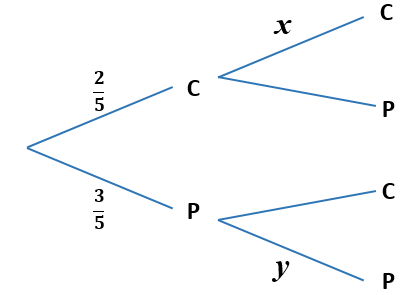(a) Write down the value of $$x$$.

(b) Write down the value of $$y$$.

(c) Find the probability that both cakes are plain.

On another shelf there are two boxes, one red and one green. The red box contains four chocolate cakes and five plain cakes and the green box contains three chocolate cakes and four plain cakes. Ben randomly chooses either the red or the green box and randomly selects a cake.

(d) Copy and complete the tree diagram below.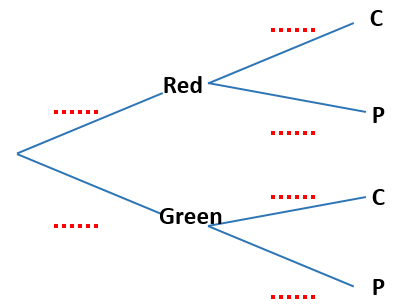(e) Find the probability that he chooses a chocolate cake.

(f) Find the probability that he chooses a cake from the red box given that it is a chocolate cake.

### 10.

IB Standard

Jane and David play two games of golf. The probability that Jane wins the first game is $$\frac56$$. If Jane wins the first game, the probability that she wins the second game is $$\frac67$$.

If Jane loses the first game, the probability that she wins the second game is $$\frac34$$

(a) Copy and complete the following tree diagram.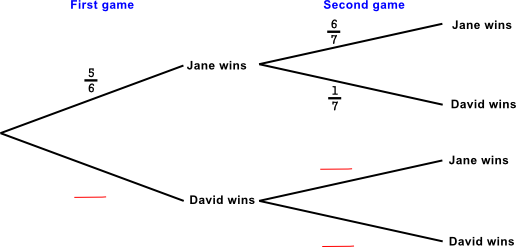(b) Find the probability that Jane wins the first game and David wins the second game.

(c) Find the probability that David wins at least one game.

(d) Given that David wins at least one game, find the probability that he wins both games.

### 11.

IB Analysis and Approaches

A factory manufactures switches. It is known that the probability that a switch is found to be defective is 0.04. A random sample of 50 switches is tested.

(a) Find the probability that there is at least one defective switch in the sample.

(b) Given that there is at least one defective switch in the sample, find the probability that there are at least three defective switches.

### 12.

A-Level

Mathsland's national currency comes in denominations of 1 unit, 5 units, 10 units and 50 units. Sofya places this collection of these coins in her purse then, without looking, takes out two coins at random, one after the other.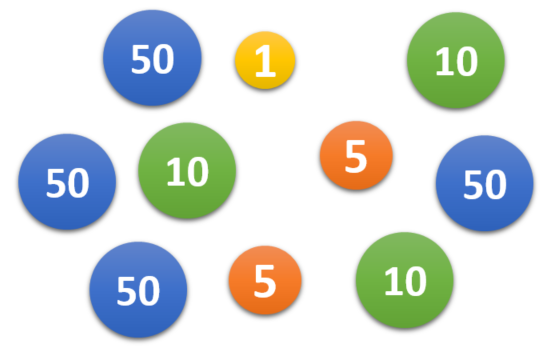Draw a tree diagram to represent the situation and then use it to calculate the probability that the second coin that Sofya takes out of her purse has a greater value than the first.

### 13.

IB Standard

Two events A and B are such that $$P(A) = 0.57$$ and $$P (A \cap B) = 0.21$$.

(a) Find $$P (A \cap B' )$$.

(b) Given that $$P((A \cup B)') = 0.17$$, find $$P(A|B')$$.

If you would like space on the right of the question to write out the solution try this Thinning Feature. It will collapse the text into the left half of your screen but large diagrams will remain unchanged.

The exam-style questions appearing on this site are based on those set in previous examinations (or sample assessment papers for future examinations) by the major examination boards. The wording, diagrams and figures used in these questions have been changed from the originals so that students can have fresh, relevant problem solving practice even if they have previously worked through the related exam paper.

The solutions to the questions on this website are only available to those who have a Transum Subscription.

Exam-Style Questions Main Page

Search for exam-style questions containing a particular word or phrase:

To search the entire Transum website use the search box in the grey area below.